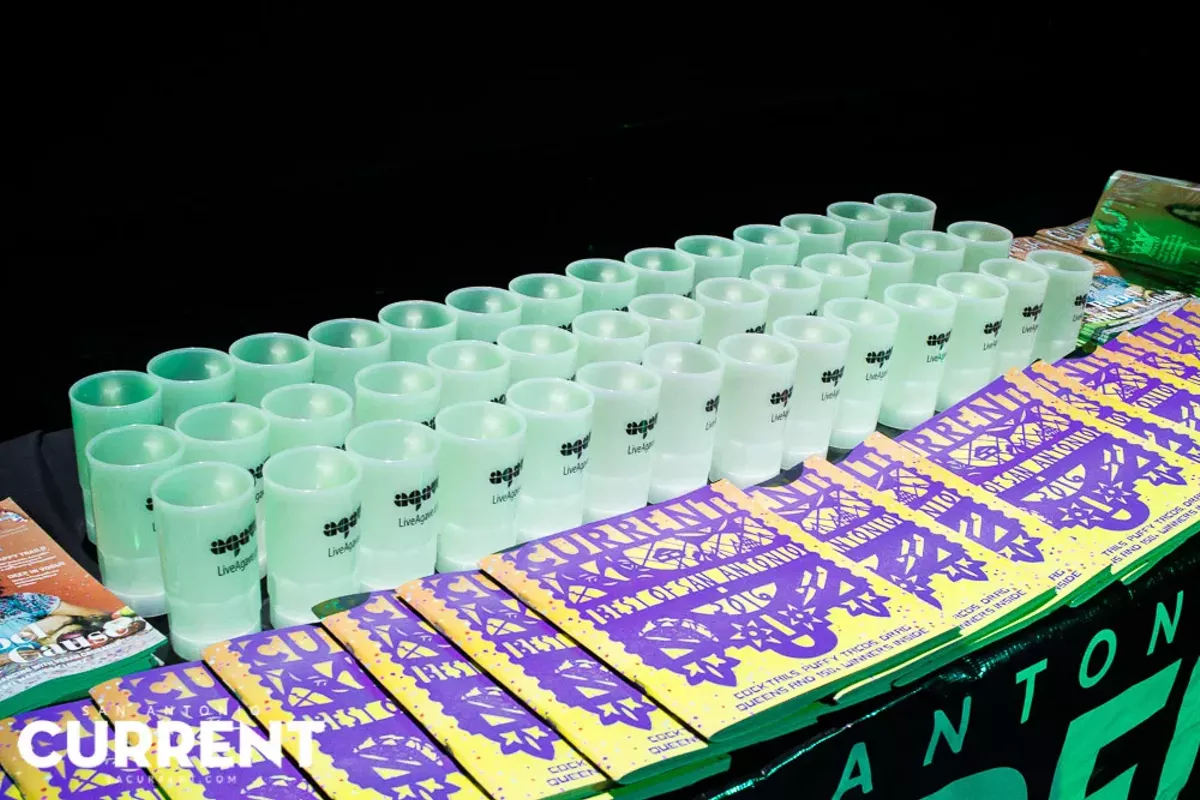# Best of San Antonio 2016: A Night of People, Performances and Partying

Tweet
Submit to Reddit
Email
The stage at the Best of San Antonio was full of people and performances. A big thanks goes to our vendors Cavender Toyota, Bucay Dermatology, Core Physique Studios, DWI Dude, UBreakiFix, and the event's vendors Botika, Cake Crumbs, Local Coffee, Lick, Fairview Coffee, Thai Topaz, Bill Miller BBQ and5 Points Local for a great night.
04/21/2016
Photos by B.K. McKee
OF 108
PREV NEXTB.K. McKee
B.K. McKee
B.K. McKee
B.K. McKee
B.K. McKee
B.K. McKee
B.K. McKee
B.K. McKee
B.K. McKee
B.K. McKee
B.K. McKee
B.K. McKee
B.K. McKee
B.K. McKee
B.K. McKee
B.K. McKee
B.K. McKee
B.K. McKee
B.K. McKee
B.K. McKee
B.K. McKee
B.K. McKee
B.K. McKee
B.K. McKee
B.K. McKee
B.K. McKee
B.K. McKee
B.K. McKee
B.K. McKee
B.K. McKee
B.K. McKee
B.K. McKee
B.K. McKee
B.K. McKee
B.K. McKee
B.K. McKee
B.K. McKee
B.K. McKee
B.K. McKee
B.K. McKee
B.K. McKee
B.K. McKee
B.K. McKee
B.K. McKee
B.K. McKee
B.K. McKee
B.K. McKee
B.K. McKee
B.K. McKee
B.K. McKee
B.K. McKee
B.K. McKee
B.K. McKee
B.K. McKee
B.K. McKee
B.K. McKee
B.K. McKee
B.K. McKee
B.K. McKee
B.K. McKee
B.K. McKee
B.K. McKee
B.K. McKee
B.K. McKee
B.K. McKee
B.K. McKee
B.K. McKee
B.K. McKee
B.K. McKee
B.K. McKee
B.K. McKee
B.K. McKee
B.K. McKee
B.K. McKee
B.K. McKee
B.K. McKee
B.K. McKee
B.K. McKee
B.K. McKee
B.K. McKee
B.K. McKee
B.K. McKee
B.K. McKee
B.K. McKee
B.K. McKee
B.K. McKee
B.K. McKee
B.K. McKee
B.K. McKee
B.K. McKee
B.K. McKee
B.K. McKee
B.K. McKee
B.K. McKee
B.K. McKee
B.K. McKee
B.K. McKee
B.K. McKee
B.K. McKee
B.K. McKee
B.K. McKee
B.K. McKee
B.K. McKee
B.K. McKee
B.K. McKee
B.K. McKee
B.K. McKee
B.K. McKee
1/108
B.K. McKee

## Join the San Antonio Current Press Club

At a time when local-based reporting is critical, support from our readers is essential to our future. Join the San Antonio Current Press Club for as little as \$5 a month.